「多元线性回归模型」非常常见，是大多数人入门机器学习的第一个案例，尽管如此，里面还是有许多值得学习和注意的地方。其中多元共线性这个问题将贯穿所有的机器学习模型，所以本文会「将原理知识穿插于代码段中」，争取以不一样的视角来叙述和讲解「如何更好的构建和优化多元线性回归模型」。主要将分为两个部分：

• 详细原理

• Python 实战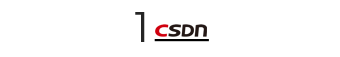### Python 实战

Python 多元线性回归的模型的实战案例有非常多，这里虽然选用的经典的房价预测，但贵在的流程简洁完整，其中用到的精度优化方法效果拔群，能提供比较好的参考价值。

#### 数据探索

``````import pandas as pd
import numpy as np
import seaborn as sns
import matplotlib.pyplot as plt

``````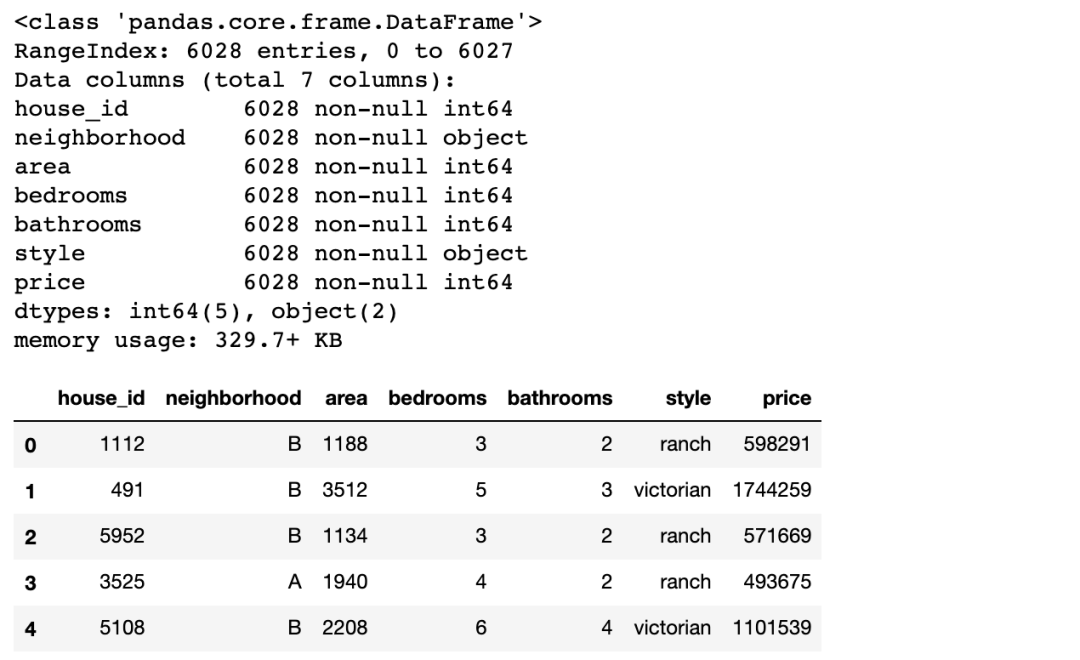• neighborhood/area: 所属街区和面积

• bedrooms/bathrooms: 卧室和浴室

• style: 房屋样式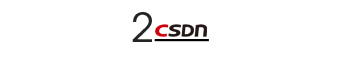#### 多元线性回归建模

``````from statsmodels.formula.api import ols
# 小写的 ols 函数才会自带截距项，OLS 则不会
# 固定格式：因变量 ~ 自变量(+ 号连接)
lm = ols('price ~ area + bedrooms + bathrooms', data=df).fit()
lm.summary()
``````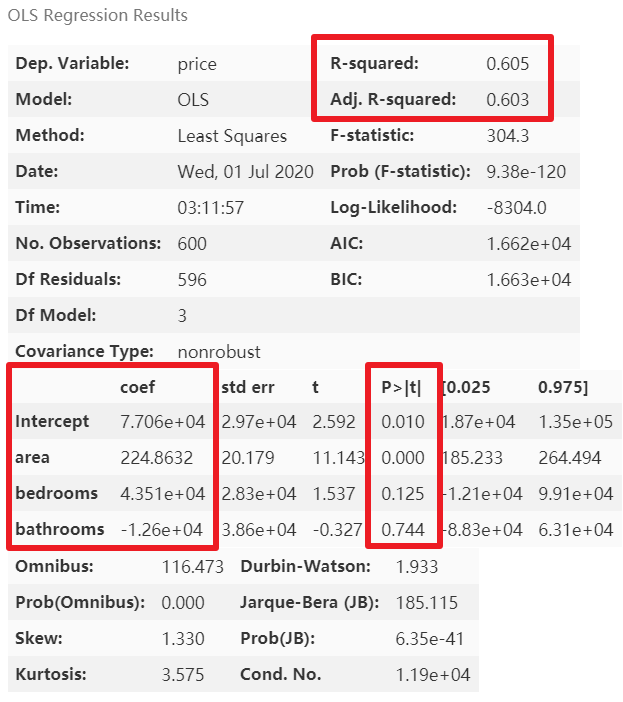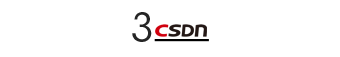#### 模型优化

``````# 类别变量，又称为名义变量，nominal variables
nominal_vars = ['neighborhood', 'style']

for each in nominal_vars:
print(each, ':')
print(df[each].agg(['value_counts']).T)  # Pandas 骚操作
# 直接 .value_counts().T 无法实现下面的效果
## 必须得 agg，而且里面的中括号 [] 也不能少
print('='*35)
``````
##### 虚拟变量的设置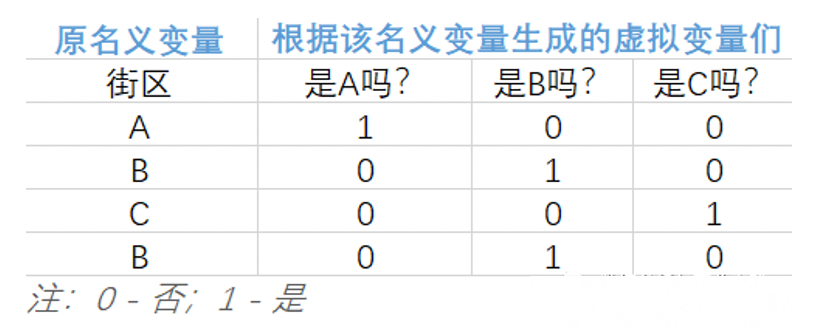• 该名义变量有 n 类，就能拆分出 n 个虚拟变量。

• 巧妙的使用 0 和 1 来达到「用虚拟变量列代替原名义变量所在类别」。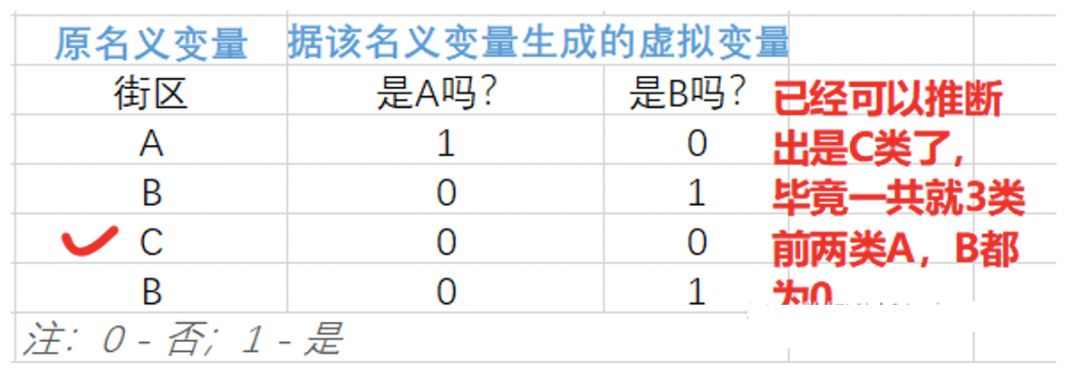• 抽出希望转换的名义变量(一个或多个)

• pandas 的 get_dummies 函数

• 与原数据集横向拼接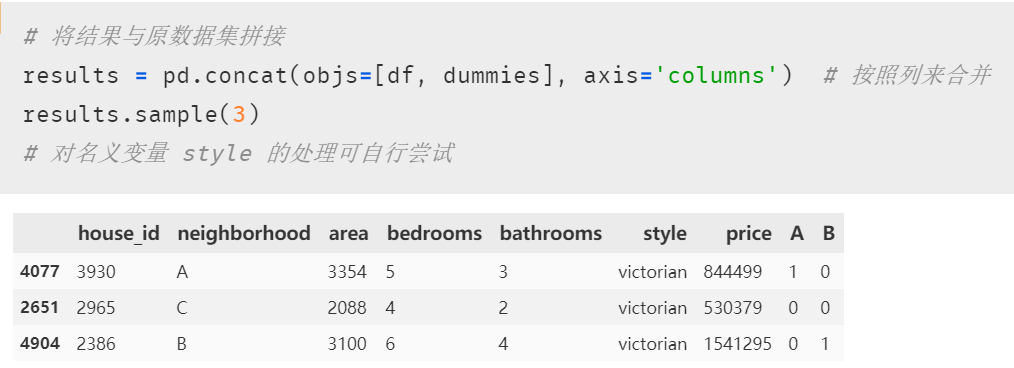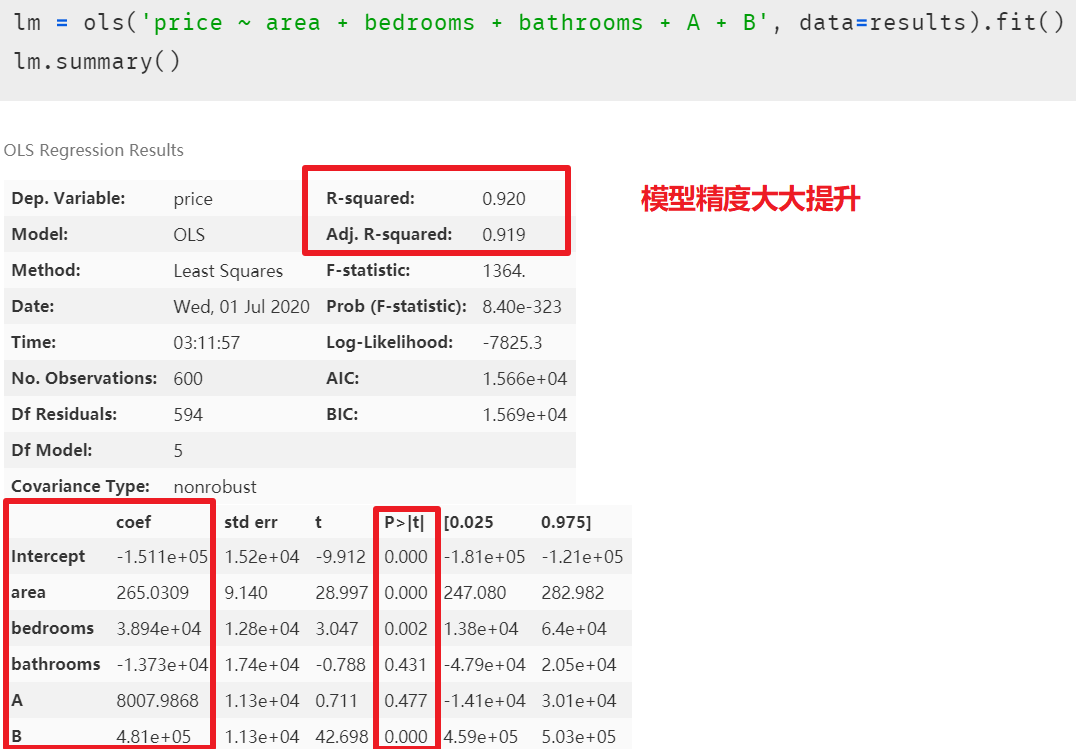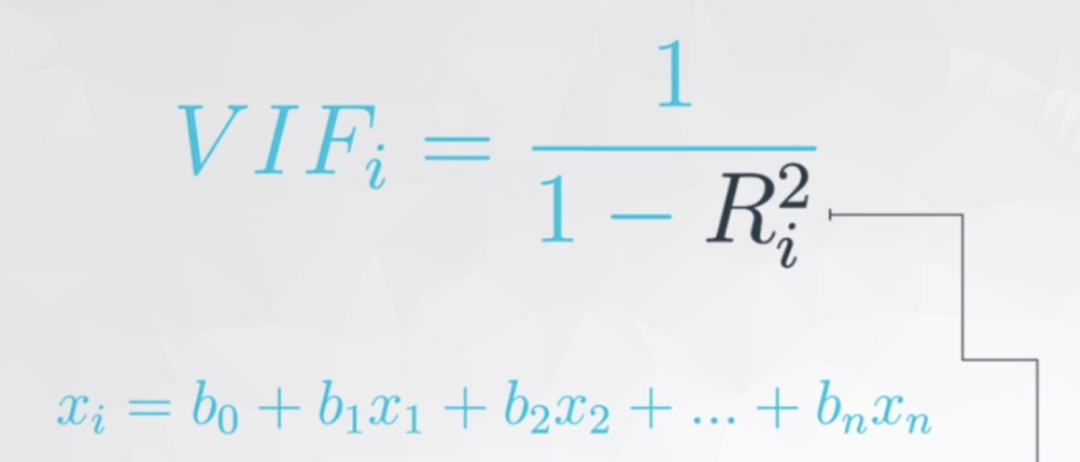• 每个自变量都会有一个膨胀因子值  ，最后根据值的大小来选择是否删减。

• 既然   表示相关性，是谁跟谁的相关性呢? 是自变量中的某一变量与除它外剩余的自变量进行多元线性回归，取回归结果，即模型精度来作为这个变量与剩余自变量的相关性。听起来可能有点绕，这里举一下实例：用 “面积、卧室数量和浴室数量” 作为自变量来预测房价，在进行自变量的方差膨胀因子的检测时，面积、卧室数和浴室数轮流做单独的因变量，剩下的两个变量作为自变量，来看看这三个自变量中哪个变量对其余两个变量的解释性高。

•    越大，如已经到了 0.9，那分母就很小，  值就等于 10，即表示这个自变量已经同时解释了另外的某个或多个自变量，存在多元共线性，可以考虑删除一些自变量。

##### 方差膨胀因子的检测

``````def vif(df, col_i):
"""
df: 整份数据
col_i：被检测的列名
"""
cols = list(df.columns)
cols.remove(col_i)
cols_noti = cols
formula = col_i + '~' + '+'.join(cols_noti)
r2 = ols(formula, df).fit().rsquared
return 1. / (1. - r2)
``````

``````test_data = results[['area', 'bedrooms', 'bathrooms', 'A', 'B']]
for i in test_data.columns:
print(i, '\t', vif(df=test_data, col_i=i))
``````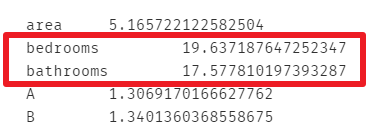``````lm = ols(formula='price ~ area + bathrooms + A + B', data=results).fit()
lm.summary()
``````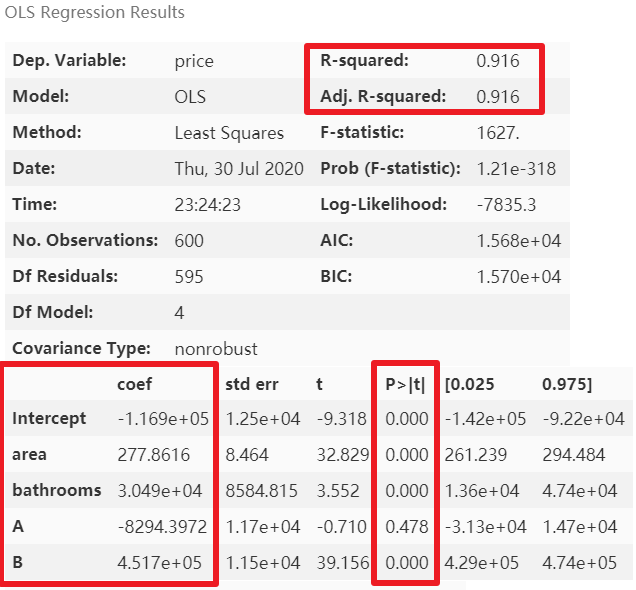``````test_data = results[['area', 'bedrooms', 'A', 'B']]
for i in test_data.columns:
print(i, '\t', vif(df=test_data, col_i=i))
``````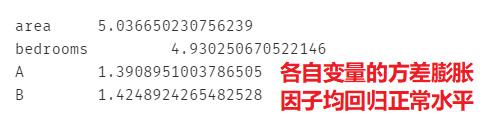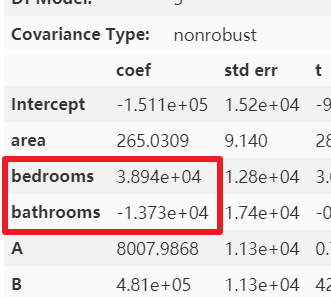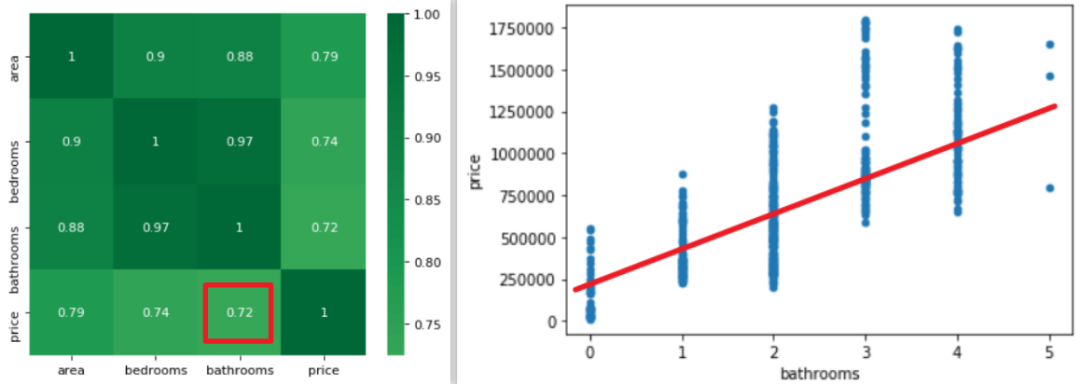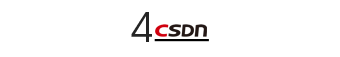#### 模型解释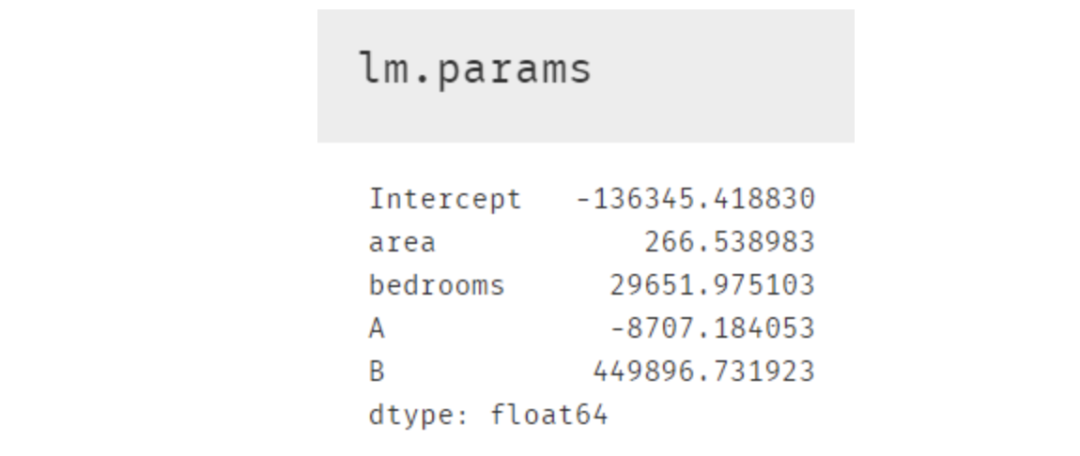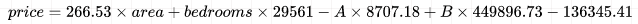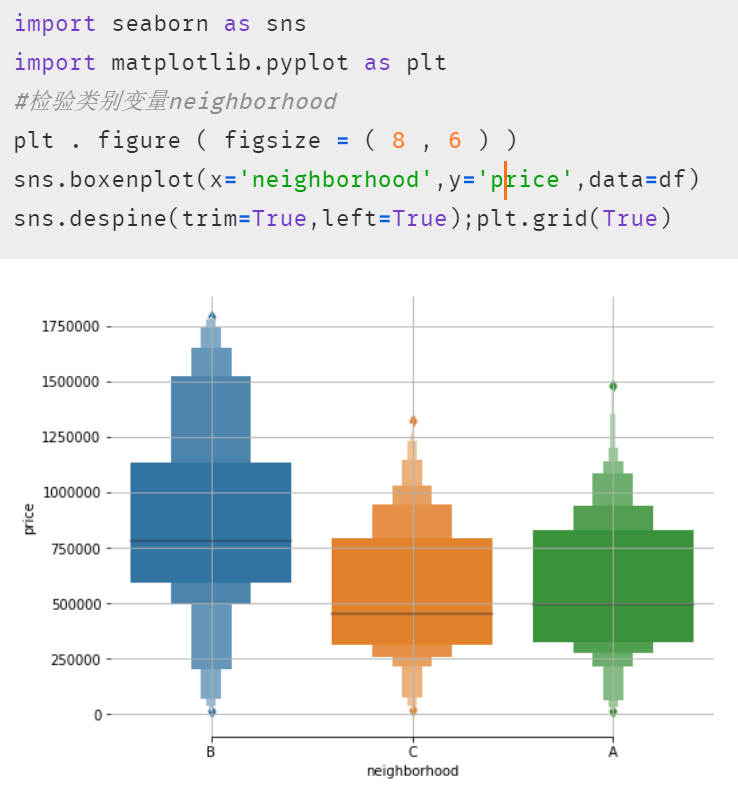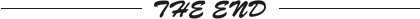``````更多精彩推荐
``````
``````☞美国禁止与字节跳动及微信交易，45 天后生效，字节跳动回应来了！
☞110+优秀作品、20+热门领域曝光， 高通如何玩转“AI 的夏天”！
☞大写“惨”？三次改变世界、却被无情出局的程序员大牛！
☞我还没考试，算法就说我的物理一定挂科
☞中台架构详解（上） | 大咖说中台
☞Eth2 验证者快速启动器发布，还有什么惊喜是我们不知道的？

``````20年前，《新程序员》创刊时，我们的心愿是全面关注程序员成长，中国将拥有新一代世界级的程序员。20年后的今天，我们有了新的使命：助力中国IT技术人成长，成就一亿技术人！